# PRICE Function In ExcelArticle byExcelMojo Team## What Is PRICE Function In Excel?

The PRICE function in Excel helps users determine the cost per \$100 face value of a bond or security, paying periodic interest. Users can apply the function when planning to borrow funds by trading bonds in place of stocks during financial analysis. Also, the function enables users to evaluate a bond’s breakeven price, provided they are aware of the specific bond’s parameters.

The PRICE function in Excel is an inbuilt financial function, so we can insert the formula from the “Function Library” or enter it directly in the worksheet.

For example, the table below contains parameter values. We will use the PRICE function in Excel to determine the price of a bond we plan to trade.

First, select cell B13, enter the formula =PRICE(B5,B6,B7,B8,B9,B10,B11), and press “Enter”.

The output is 92.1469722073499, as shown above. And once we set the data format of the target cell as Currency, the value gets rounded off to two decimal places, \$92.15.

###### Key Takeaways
• The PRICE function in Excel calculates the price per \$100 original value of a bond, paying regular interest.
• Users will find the PRICE() useful while performing financial analysis. For example, an apt scenario is when they plan to borrow capital by trading bonds in place of stocks. Also, if we know a bond’s parameters, we can apply the PRICE() to determine the specific bond’s breakeven price.
• The frequency argument can take the values 1, 2, or 4, representing annual, semi-annual, and quarterly coupon payment frequency, respectively. On the other hand, the argument basis can take a value from 0 to 4, with each value representing a day count basis type.

### PRICE() Excel Formula

The syntax of the PRICE Excel formula is:

The arguments of the PRICE Excel formula are:

• settlement: The settlement date or the coupon purchase date of the bond. So, the settlement argument is the date falling after the issue date, when the specific bond is sold to the buyer. It is a mandatory argument.
• maturity: The maturity date of the bond. In other words, it is the date the bond expires. It is a mandatory argument.
• rate: The annual coupon rate of the bond. It is a mandatory argument.
• yld: The annual yield of the bond. It is a mandatory argument.
• redemption: The redemption value for each \$100 face value of the bond. It is a mandatory argument.
• frequency: The count of coupon payments each year. It is a mandatory argument.
• basis: The day count basis type utilized by the bond. It is an optional argument. If not provided, the function takes the default value as 0.

Also, please refer to the below tables for entering the frequency and basis argument values.

### How To Use PRICE Excel Function?

We can use the PRICE function in Excel in 2 methods, namely,

1. Access from the Excel ribbon.
2. Enter in the worksheet manually.

#### Method #1 – Access from the Excel ribbon

First, choose an empty cell → select the “Formulas” tab → go to the “Function Library” group → click the “Financial” option drop-down → select the “PRICE” function, as shown below.

The “Function Arguments” window appears. Enter the argument values in the Settlement, Maturity, Rate, Yld, and Redemption fields → click “OK”, as shown below.

#### Method #2 – Enter in the worksheet manually

First, confirm whether all the PRICE() argument values required to determine the specific bond price are accurate and in the correct data formats.

1. Select an empty cell for the output.
2. Type =PRICE in the cell. [Alternatively, type =P or =PR and double-click the PRICE function from the Excel suggestions.]
3. Enter the arguments as cell references or cell values and close the brackets.
4. Press “Enter” to view the bond price.
5. Finally, set the data format of the output cell as Currency to view the result as a dollar value.

Let us take an example to understand this function.

We will calculate the price of a specific bond using PRICE function in Excel.

The table below contains the argument values required to calculate the price of a specific bond.

[Note: The first two arguments are date values, the third and fourth arguments are percentages, the fifth one is a currency value, and the last two arguments are numbers.]

The steps to calculate the bond price using PRICE function in Excel are,

1. Select cell B13, enter the formula =PRICE(C5,C6,C7,C8,C9,C10,C11), and press Enter.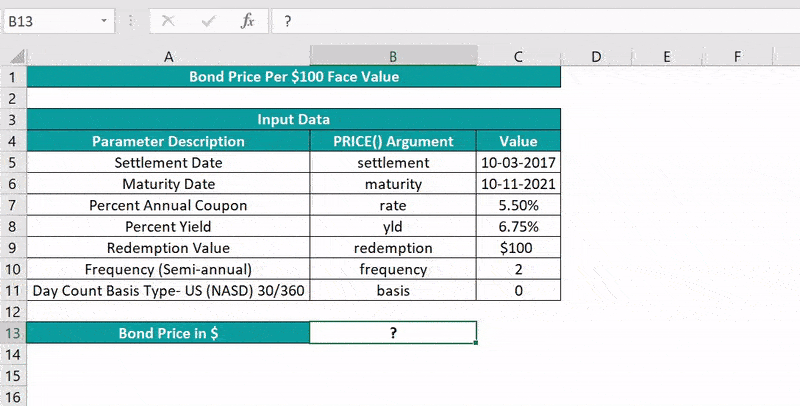2. Select cell B13 and set the Number Format in the Home tab as Currency.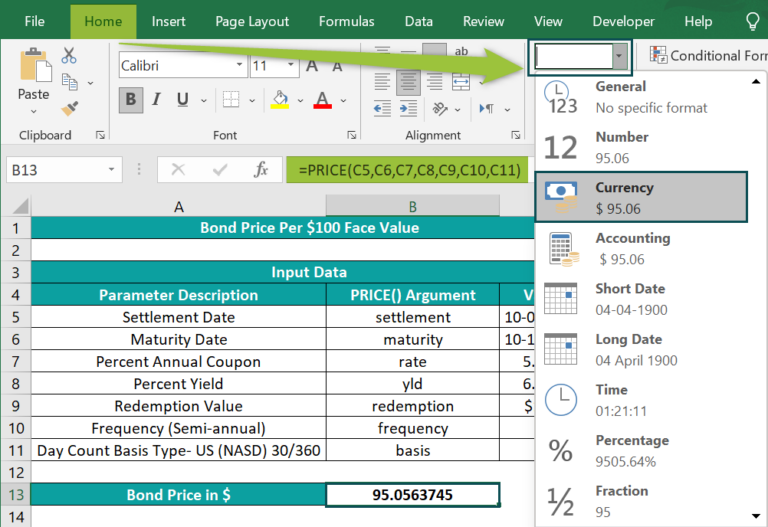The output is shown below.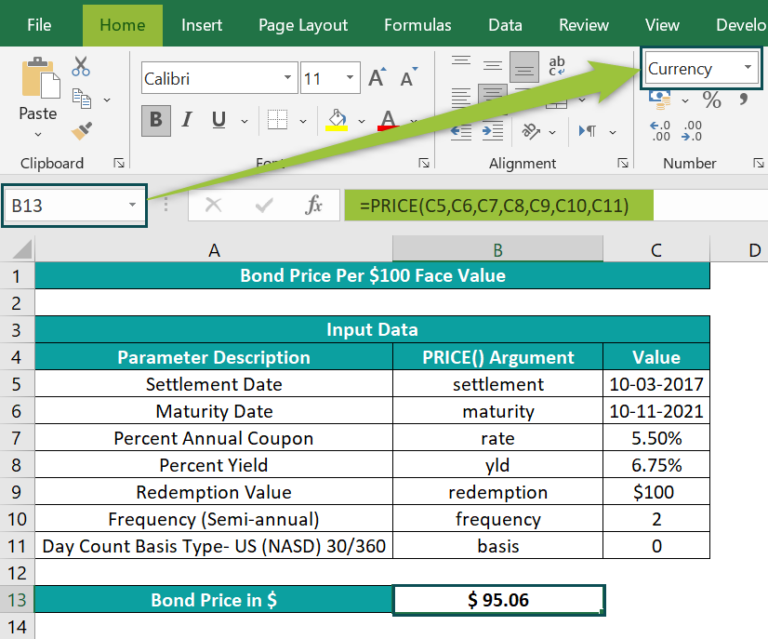[Alternate methods to derive the same results are:

Alternate Method 1 – We can enter the values directly using the PRICE() and DATE() functions. The steps are as follows:

• Step 1: Select cell B13, enter the formula =PRICE(DATE(

[It is best to enter the date values using the DATE excel function to avoid invalid dates and then the rate and redemption values, separated by commas. Next, Excel will show a drop-down list to choose the frequency argument. And once we double-click the required option to select the frequency and then enter a comma, we will see another drop-down list to enter the basis argument.]

• Step 2: In continuation with the formula, enter the values according to the parameters. The formula will now be =PRICE(DATE(2017,3,10),DATE(2021,11,10),5.5%,6.75%,100,
• Step 3: In continuation with the formula, enter the last argument as 0. The formula now is =PRICE(DATE(2017,3,10),DATE(2021,11,10),5.5%,6.75%,100,2,0
• Step 4: Close the brackets, and press “Enter”.

The output is shown below.

Alternate Method 1 – can use the formula from the Formulas tab. The steps are as follows:

• Step 1: Select cell B13 → click FormulasFinancialPRICE to open the Function Arguments window.
• Step 2: Enter the PRICE() argument values in the Function Arguments window, as shown in the image below.

And finally, click OK in the Function Arguments window, and we get the result in cell B13.

In the above example, the frequency argument is 2, as the coupon payments frequency is semi-annual.

Thus, the PRICE() returns the value 95.0563745 as the bond price. And once we set the data format of the value as Currency, we get the required result as a dollar value, \$95.06.]

### Examples

We will understand some advanced scenarios using the PRICE function in Excel examples.

#### #Example 1

We will calculate the corporate bond price, with a face value of \$100, using the PRICE function in Excel.

The below table contains all the inputs required to perform the calculation.

The steps to apply the PRICE function in Excel are,

• Step 1: Select cell B11, enter the formula =PRICE(C2,C3,C4,C5,C6,C7,C8), and press Enter. The output is shown below.
• Step 2: Select cell B11 and set the Number Format in the Home tab as Currency.

#### #Example 2

We will use the PRICE function to calculate the cost of a fixed-income security, which are typically government or corporate bonds with lower annual coupon rates and yield percentages.

The below table contains the inputs required to determine the cost of a fixed-income security.

The steps to calculate the cost using the PRICE function in Excel are,

• Step 1: Select cell B11, enter the formula =PRICE(C2,C3,C4,C5,C6,C7,C8), and press Enter.

Step 2: Select cell B11 and set the Number Format in the Home tab as Currency.

The output is \$80.47, as shown above. Here, the frequency of coupon payments each year is quarterly. Therefore, the frequency argument is 4.

#### #Example 3

Let us see how the PRICE function in Excel behaves when the argument values are incorrect.

• #VALUE! Error.
• #NUM! Error.
##### #VALUE! Error
• Scenario 1 – The table below contains the PRICE() arguments required to calculate the price of a bond to be allocated. And cell B11 is the target cell containing the PRICE(). However, the output in cell B11 returns a #VALUE! error as the value of the settlement argument is an invalid date.
• Scenario 2 – In this case, the PRICE() output is the #VALUE! error because the argument basis is a non-numeric value, as shown below.
##### #NUM! Error

The following images show the various situations where the PRICE() can return the #NUM! error.

• Scenario 1 – The settlement date falls after the maturity date, leading to the #NUM! error, as shown below.
• Scenario 2 – The rate value is less than 0, which results in the #NUM! error, as shown below.
• Scenario 3 – The redemption value is \$0, resulting in an error, as shown below.
• Scenario 4 – The frequency argument is not 1, 2, or 4, causing the PRICE() to return the error, as shown below.
• Scenario 5 – The basis argument is not 0, 1, 2, 3, or 4, leading to the error, as shown below.

Once we correct the above errors the PRICE() will work perfectly, and give accurate results, as shown below.

### Important Things To Note

• When supplying the settlement and maturity date values directly to the PRICE function in Excel, use the DATE() to avoid entering invalid dates.
• If the supplied frequency or basis arguments are not integers, or the settlement or maturity dates are serial numbers but not integer values. Then such values get truncated.
• For invalid dates and non-numeric argument values, the PRICE() returns the #VALUE! error.
• Suppose the settlement date is greater than the maturity date, the yld or rate arguments are less than zero, or the redemption argument is less than or equal to zero. On the other hand, the frequency argument is not 1, 2, or 4, or the basis argument is not 0, 1, 2, 3, or 4. Then, in such cases, the PRICE() output is the #NUM! error.

1. Where is the PRICE function in Excel?

The PRICE function is in the Formulas tab.

First, choose an empty cell → select the “Formulas” tab → go to the “Function Library” group → click the “Financial” option drop-down → select the “PRICE” function, as shown below.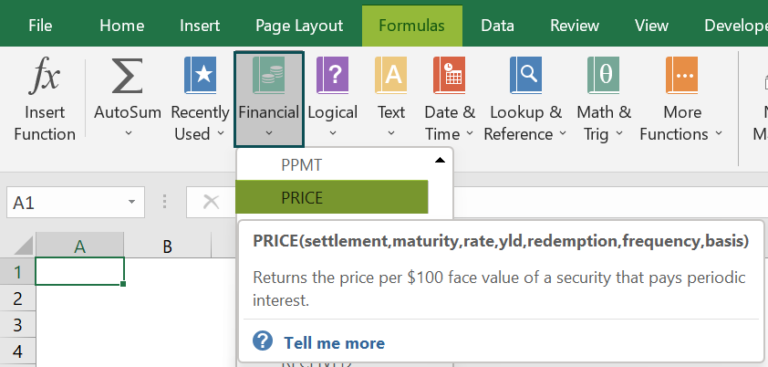2. How to apply the PRICE function in Excel VBA?

We can apply the PRICE function in Excel VBA using the following method:

Application.WorksheetFunction.Price(settlement,maturity,rate,yld,redemption, frequency,basis)

For example, the table below contains the inputs required to determine a bond’s price per \$100 face value.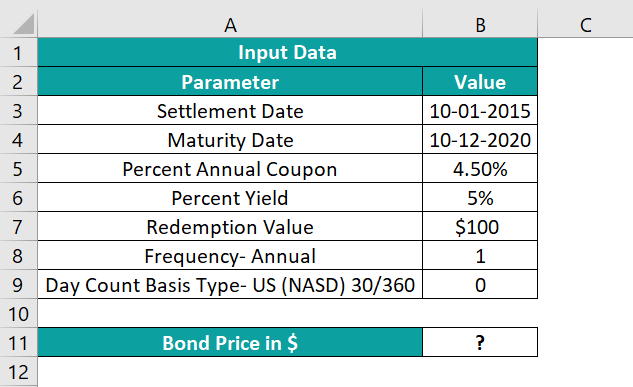The steps to apply the PRICE function in Excel and VBA are as follows:

• Step 1: Select cell B11, and set the Number Format in the Home tab as Currency.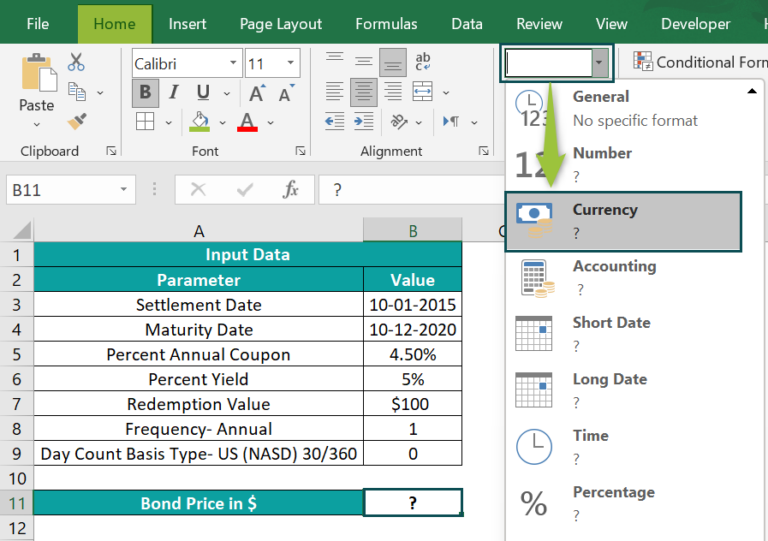• Step 2: In the worksheet with the above table, press the keys Alt + F11 to open the VBA Editor.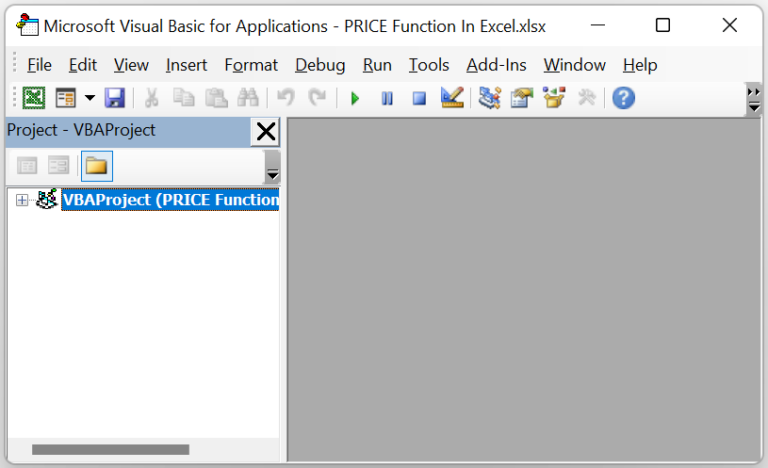• Step 3: Choose the required VBAProject and click InsertModule to open a new module window, Module1.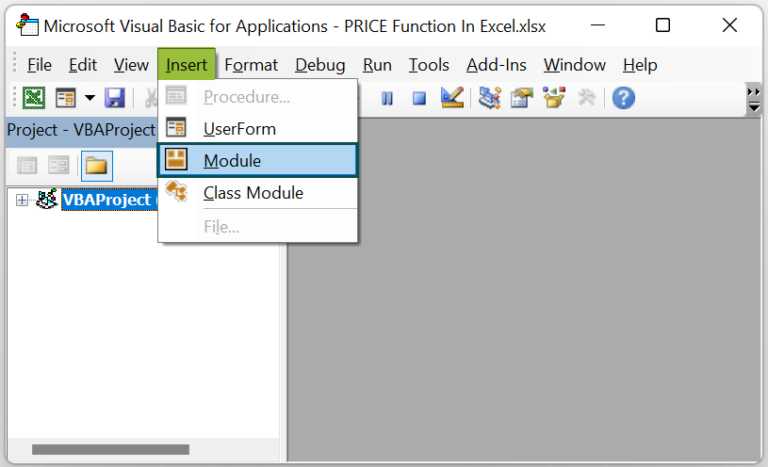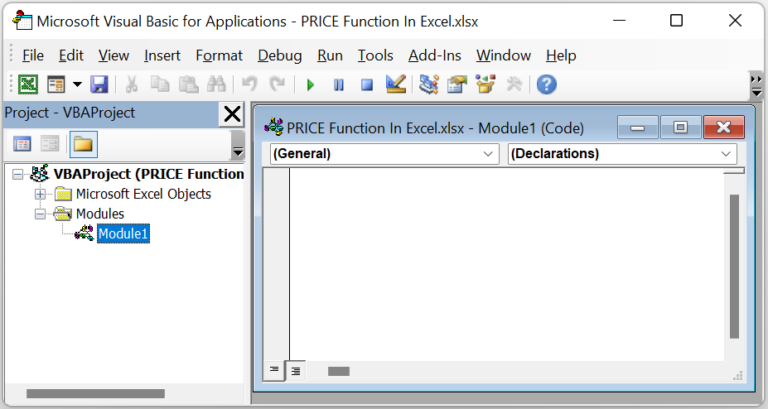• Step 4: Enter the VBA code, as shown in the below image, in the Module1 window to execute the PRICE().

Sub PRICE_fn()
Range(“B11”) = Application.WorksheetFunction.Price(Range(“B3”), Range(“B4”), Range(“B5”), Range(“B6”), Range(“B7”), Range(“B8”), Range(“B9”))
End Sub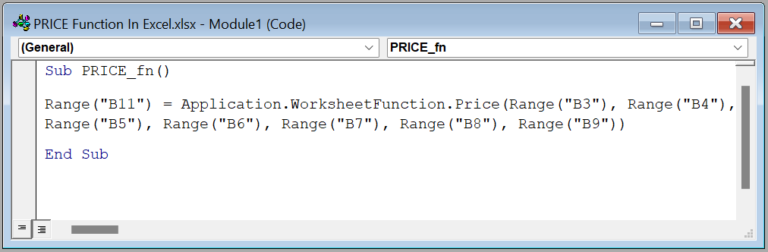• Step 5: Click the Run Sub/UserForm icon in the top menu to run the commands in the Module1 window.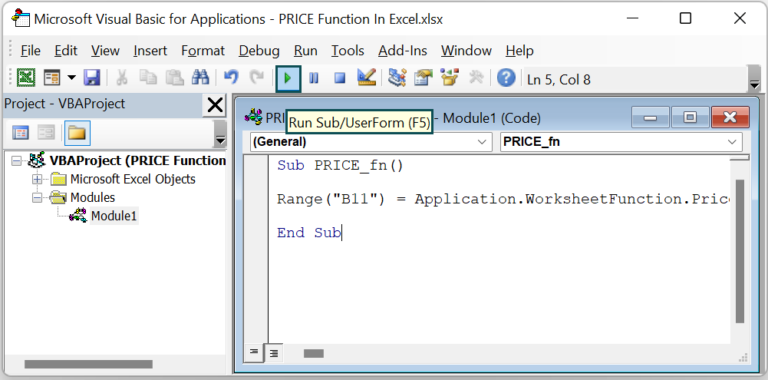Now, we can open the worksheet to view the PRICE() output in the target cell B11.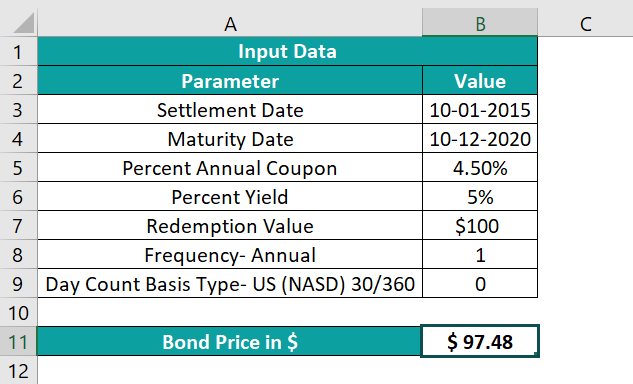3. What are the uses of the PRICE function in Excel?

The uses of the PRICE function in Excel are:
It helps calculate a corporate bond’s price per \$100 face value. We can also use it to determine the cost of a fixed-income security.
It is useful for evaluating a bond’s clean or quoted price, a price that excludes accrued interest.